﻿ Simulation of Three-mass Mechanical System using MATLAB Software

### Simulation of Three-mass Mechanical System using MATLAB Software

Marek Moravič, Oskar Ostertag, Darina Hroncová

Journal of Automation and Control

## Simulation of Three-mass Mechanical System using MATLAB Software

Marek Moravič1,, Oskar Ostertag2, Darina Hroncová3

1Department of Construction, Automotive and Transport Engineering, Faculty of Mechanical Engineering, Technical University of Košice, Košice, Slovak republic

2Department of Applied Mechanics and Mechanical Engineering, Faculty of Mechanical Engineering, Technical University of Košice, Košice, Slovak republic

3Department of Mechatronics, Faculty of Mechanical Engineering, Technical University of Košice, Košice, Slovak republic

### Abstract

The aim of the paper is the dynamic analysis of selected models of running gear by using MATLAB/Simulink. This paper concerns with numerical approach to a vehicle vertical vibration based on kinematic excitation. Kinematic variables calculation is demonstrated on the quarter three-mass dynamic model with additional shock absorber and spring. There is mathematical model that is numerically solved in MATLAB and is illustrated here together with the simulation in Simulink by block scheme. The results could be used for teaching purposes.

• Marek Moravič, Oskar Ostertag, Darina Hroncová. Simulation of Three-mass Mechanical System using MATLAB Software. Journal of Automation and Control. Vol. 3, No. 3, 2015, pp 114-117. http://pubs.sciepub.com/automation/3/3/15
• Moravič, Marek, Oskar Ostertag, and Darina Hroncová. "Simulation of Three-mass Mechanical System using MATLAB Software." Journal of Automation and Control 3.3 (2015): 114-117.
• Moravič, M. , Ostertag, O. , & Hroncová, D. (2015). Simulation of Three-mass Mechanical System using MATLAB Software. Journal of Automation and Control, 3(3), 114-117.
• Moravič, Marek, Oskar Ostertag, and Darina Hroncová. "Simulation of Three-mass Mechanical System using MATLAB Software." Journal of Automation and Control 3, no. 3 (2015): 114-117.

 Import into BibTeX Import into EndNote Import into RefMan Import into RefWorks

123
Prev Next

### 1. Introduction

Method of releasing is applied to drawing up mathematical model. Kinetic equations are drawn up by Newton’s method. The result is kinematic quantities graphically represented and their numerical values. Selecting the way of solution is influenced by concern about mechanical system vibration problems . MATLAB is efficient, interactive setting for scientific and engineering calculations. To be able to solve in Matlab it is necessary to modify system of differential equations of second order to system of differential equations of first order. Detailed calculation process is introduced in work .Download asVeiw figureFigures index
Figure 1. Quarter three-mass model

### 2. Drawing up Mathematical Model by Method of Releasing

Calculation of kinematic quantities is demonstrated on quarter three-mass dynamic model with additional damper and spring (Figure 1) [2, 3]. The system is solved in MATLAB/Simulink.Download asVeiw figureFigures index
Figure 2. Released quarter model

Running gear is kinetically excited. Kinetic equations of mechanical system are made up by applying of releasing method (Figure 2).

Kinetic equations of damped three-mass mechanical system (Figure 2) are composed by using of releasing method and look like: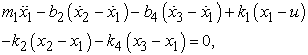(1)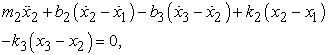(2)(3)

We put the substitution: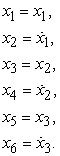(4)

By derivation equations (4) according to time we achieve:(5)

By repeated using of equations (4) and modifying we achieve six differential equations of first order: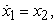(6)(7)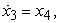(8)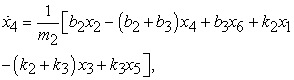(9)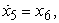(10)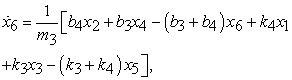(11)

### 3. Solution of Mechanical System Vertical Vibration Using MATLAB/Simulink Program

At first the entry has to be defined – course of obstacle for solution in MATLAB. The function is(12)

where A is obstacle height, L0 is its length and v is car speed .

The equations (6), (7), (8), (9), (10) and (11) need to be transcribed to m-file of MATLAB (Table 1). The name of file will be saved according to name of function. For numerical solution of differential equations MATLAB applies predefined functions that differ about method of integration. The time course depiction can be gained by standard function ode45 (Figure 3) .

#### Table 1. M-files of MATLABDownload asVeiw figureFigures index
Figure 3. Displacements and velocities depiction

Modeling of mechanical system in Simulink helps us graphically represent the time progressions of kinetic and dynamic quantities. Modifying derived kinetic equations (1), (2) and (3) for three-mass model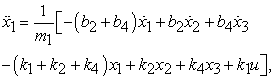(13)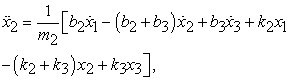(14)(15)

we make block diagram (Figure 4).Download asVeiw figureFigures index
Figure 4. The block diagram of three-mass system in Simulink

Table 2 shows blocks overview from Figure 4, but the library in Simulink contains also other usable blocks for purposes of problem solving.

#### Table 2. Used blocks in program Simulink

There is a recommendation to change primal value 1e-3 to 1e-15 in main menu Simulation, Configuration Parameters before launching a simulation. There is also an option to set time lag of simulation.

The course of obstacle was made up by block Signal Builder. This course needs to be exported File, Export to Workspace and then Start simulation needs to be launched (Figure 5) .Download asVeiw figureFigures index
Figure 5. Block Signal Builder

In Figure 6 there is an illustration of defining random excitation in block Signal Builder.Download asVeiw figureFigures index
Figure 6. The illustration of defining random excitation in block Signal Builder

Table 3 shows the forms of input data recording to start a simulation in Simulink. These data will be saved into Workspace. It is recommended to use m-file for purposes of faster input editing.

#### Table 3. The forms of input data recording in Simulink

Graphical course of quantities is represented by m-file by subplot and plot (Figure 7, Figure 8). Function Plot is entered in Table 4.Download asVeiw figureFigures index
Figure 7. Displacement, velocity and acceleration course of mass 1 next to each other – simout1

#### Table 4. Function PlotDownload asVeiw figureFigures index
Figure 8. Displacement depiction of mass 3 only – simout5

### 4. Conclusion

The methodology of three-mass system calculation being solved by deriving of kinetic equations needed for numerical solution in MATLAB is illustrated here together with the simulation in Simulink by block schemes. First-order differential equations need to be assigned for solution in MATLAB and these equations are obtained from modification of second-order differential equations. Making up scheme in Simulink needs to proceed from second-order differential equation that describes system performance.

### Acknowledgement

This contribution is a result of the project Slovak Grant Agency – project VEGA No. 1/0937/12 “Development of non-traditional experimental methods for mechanical an mechatronical systems”, project KEGA No. 048TUKE-4/2014 “Increasing of knowledge base of students in area of application of embedded systems in mechatronic systems” and project VEGA No. 1/1205/12 “Numerical modeling of mechatronic systems”.

### References

  Hassaan, G., A., “Car Dynamics using Quarter Model and Passive Suspension, Part I: Effect of Suspension Damping and Car Speed,” in International Journal of Computer Techniques, 1(2). 1-9. 2014.In article  Moravič, M., Computer Modelling of the Suspension of the Running Gear (in Slovak), Diploma thesis, TU of Košice, Faculty of Mechanical Engineering, 2013.In article  Segľa, Š., Reich, Š., “Optimization of parameters of a semi-active suspension and its comparison with active and passive suspensions”, in Dynamika tuhých a deformovatelných těles 2006, Ústí nad Labem, 183-190.In article  Ostertag, O., Fabian, M., Moravič, M., Filičko, J., Boslai, R., “Řešení mechanismu kol s integrovaným elektromotorem, elektronickou brzdou, pružením a zavěšením,” in Cad, 24(5). 30-33. 2014.In article  Hroncová, D., “Riešenie mechanických sústav v prostredí MATLAB, Simulink a SimMechanics”, in Dynamika tuhých a deformovatelných těles 2008, Ústí nad Labem, 69-74.In article